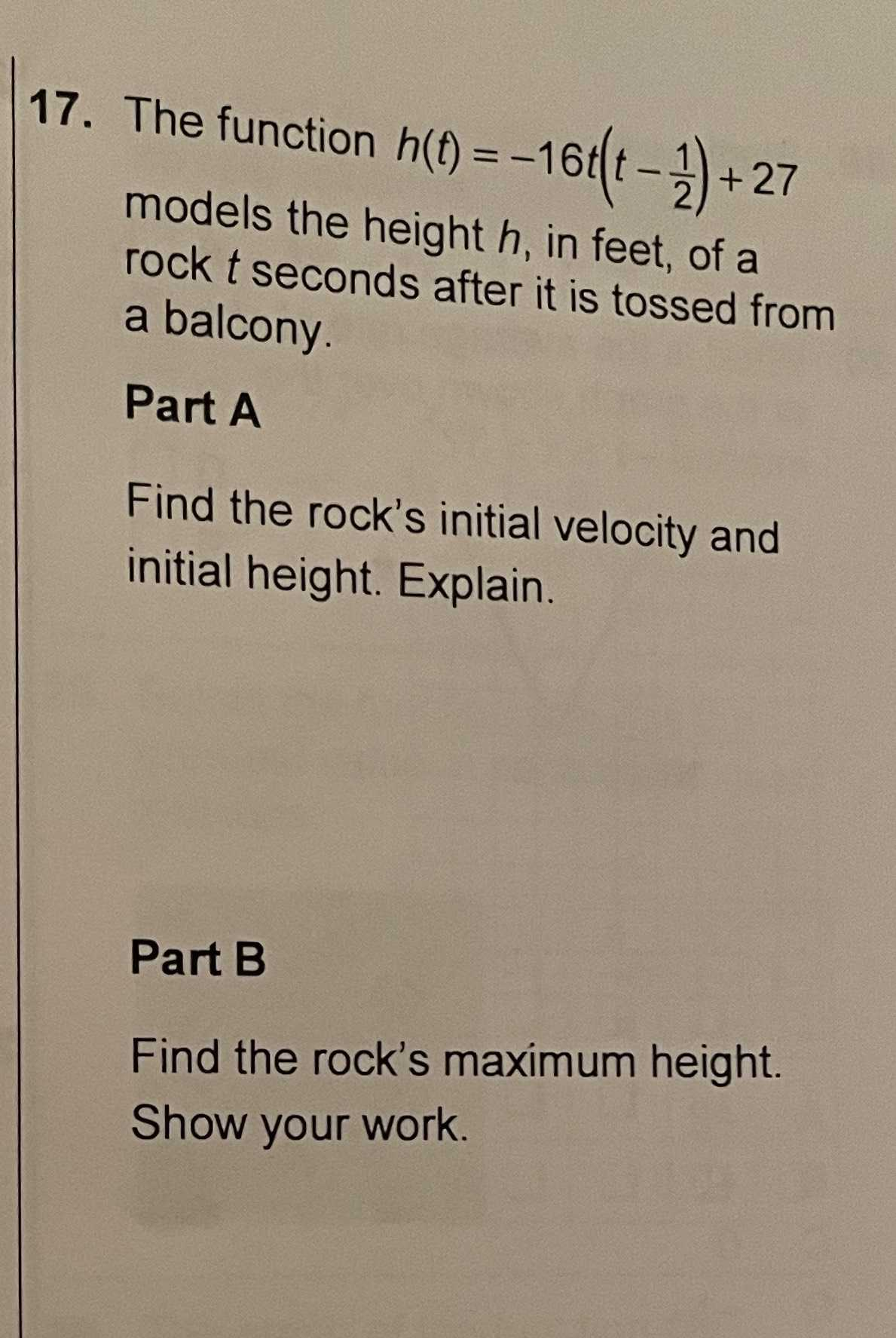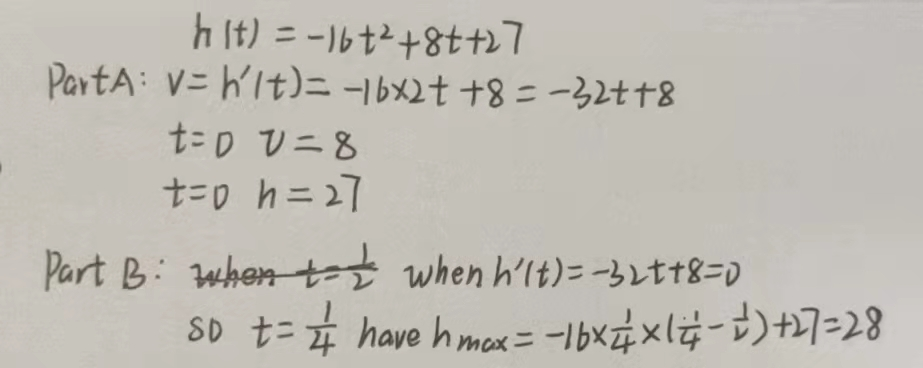### ¿Todavía tienes preguntas de matemáticas?

Pregunte a nuestros tutores expertos
Algebra
Pregunta17. The function $$h ( t ) = - 16 t ( t - \frac { 1 } { 2 } ) + 27$$

models the height $$h$$ , in feet, of a rock $$t$$ seconds after it is tossed from a balcony. Part A Find the rock's initial velocity and initial height. Explain. Part B Find the rock's maximum height. Show your work.